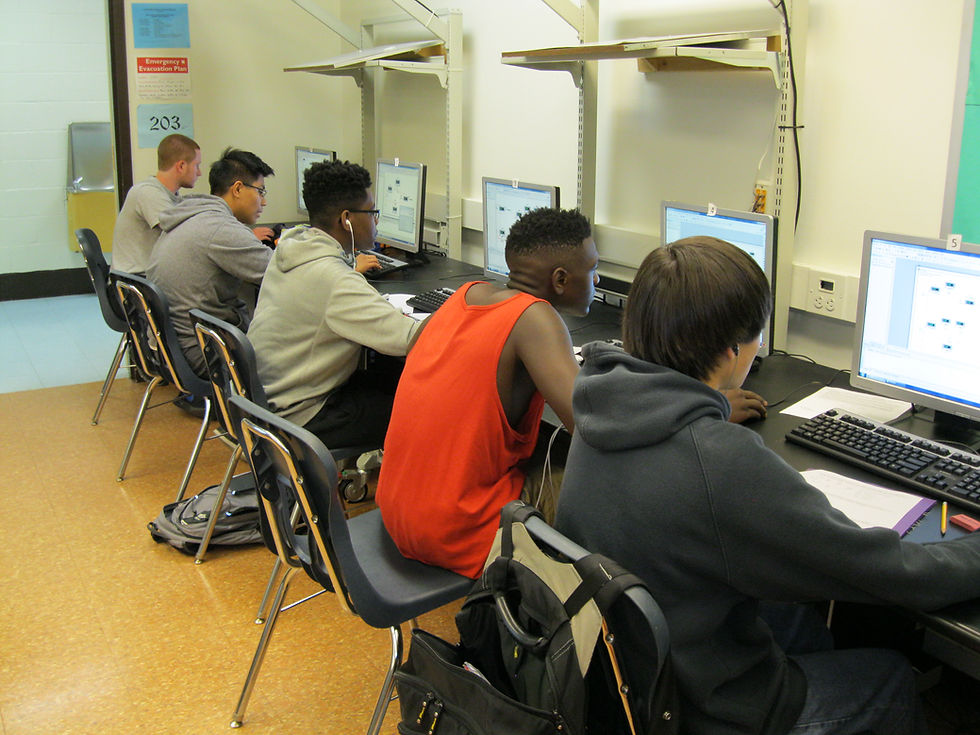top of page
Search
• AACPS DoDEA

# Computer Simulation of Ohm's Law

Have you ever wondered what happens when you plug too many things into the same power strip? We can probably guess - and be right - that it would "flip" the breaker in our house. However, why does it do that? What are the electrical principles behind it?It is these types of questions that are at the crux of the Electricity unit at Meade High during an ordinary Technology Education class. The term ordinary probably doesn't mean the same thing if your memories of school involved desks and chalk boards. The new ordinary involves hands on activities, electrical simulations and "real world" application.

During this particular lesson, the students are using a computer simulator to arrange circuit components that are connected to a theoretical DC power source – your home has AC power. These students are learning about Ohm’s Law in an electrical circuit and calculating resistance, current and voltage.It is during this calculating that the students apply algebraic skills to engineering. Ohm's Law uses the formula I=V/R (I is current, V is volts, R is resistance). In the simulation the students are given two of the values and must calculate the third.

While this particular lesson only scratches the surface of electrical circuits, the concept of Ohm's Law has far reaching applications. The same law can be applied to electronics, automotive systems, and even basic home repair. While not every student in the